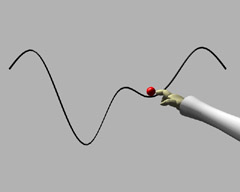# Touching Soap Films

An Introduction to Minimal Surfaces
By Hermann Karcher and Konrad Polthier

Up
Prev
Next

## Geometric Properties IFig 7. Energy levels for a 1-dimensional curve.

Let us examine area-minimizing surfaces a little more closely. Obviously the outer surface of every mountain top is larger than the surface on the ground underneath the mountain, and therefore the surface area would be reduced if the mountain were removed. Meusnier, pursuing this argument - that a minimal surface cannot exhibit any domes but on the contrary must at every point be curved toward both sides - concluded that in a small neighborhood of every point, the surface must resemble a horse's saddle, which curves upward in the longitudinal direction and downward in the transverse direction. An exact analysis shows that both curvatures must be of equal magnitude.

In order to make these curvature properties more precise, we must first clarify what we mean by the curvature of a curve in the plane. A large circle is more weakly curved than a small circle. The mathematical definition corresponds to this intuitively obvious representation of curvature. A circle of radius r is assigned a curvature equal to 1/r. A straight line, considered as the limit of larger and larger circles, has curvature zero.

A circle is equally curved at every one of its points. On the other hand, for an arbitrary curve, the curvature varies from point to point, and yet we are led back to the circle. Let us consider a car driving along such a curve drawn on the surface of an empty parking lot.

The positions of the wheels of the car at every point determine a circle on which the car would continue to travel - the so-called circle of curvature - if the driver did not continuously change the position of the steering wheel. The curvature at each point on the curve is defined as the curvature of its associated circle.